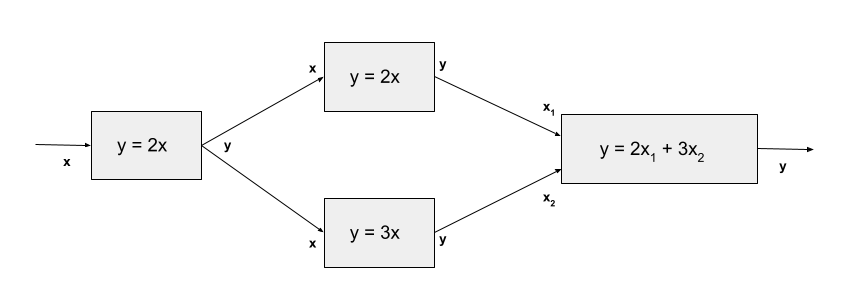# Using OpenMDAO with MPI#

In the feature notebooks for ParallelGroup and for Distributed Variables, you learned how to build a model that can take advantage of multiple processors to speed up your calculations. This document gives further details about how data is handled when using either of these features.

## Parallel Subsystems#

The ParallelGroup allows you to declare that a set of unconnected Components or Groups should be run in parallel. For example, consider the model in the following diagram:This model contains two components that don’t depend on each other’s outputs, and thus those calculations can be performed simultaneously by placing them in a ParallelGroup. When a model containing a ParallelGroup is run without using MPI, its components are just executed in succession. But when it is run using mpirun or mpiexec, its components are divided amongst the processors. To take fullest advantage of the available processors, the number of subsystems in the ParallelGroup should be equal to the number of available processors. However, it will still work if the number of processors is higher or lower than you need. If you don’t give it enough processors, then some processors will sequentially execute some of the components. If the number of subsystems is evenly divisible by the number of processors, then you won’t have idle time on any of the processors, so that is ideal. If you give your model too many processors, those processors will be idle during execution of the parallel subsystem.

The following diagram shows the same example executing on 2 processors.We see here that every component that isn’t under the ParallelGroup is executed on all processors. This is done to limit data transfer between the processors. Similarly, the input and output vectors on these components is the same on all processors. We sometimes call these duplicated variables, but it is clearer to call them non-parallel non-distributed variables.

In contrast, the inputs and outputs on the parallel components only exist on their execution processor. In this model, there are parallel outputs that need to be passed downstream to the final component. To make this happen, OpenMDAO broadcasts them from the rank that owns them to every rank. This can be seen in the diagram as the crossed arrows that connect to x1 and x2.

Since component execution is repeated on all processors, component authors need to be careful about file operations which can collide if they are called from all processors at once. The safest way to handle these is to restrict them to only write files on the root processor. In addition, the computation of derivatives is duplicated on all processes except for the components in the parallel group, which handle their own unique parts of the calculation. However this is only true in forward mode.

## Reverse-mode Derivatives in Parallel Subsystems#

Reverse-mode derivative calculation is the single exception where the computation on non-parallel, non-distributed portions is different on each processor. This can cause confusion if you are, for example, printing the values of the derivatives vectors when using the matrix-free API.

To understand what is happening, let’s examine how derivatives are computed in reverse mode for the example used above.In this diagram, our model has one derivative to compute. We start with a seed of 1.0 in the output, and propagate that through the model (as denoted by the red arrows), multiplying by the sub-jacobians in each component as we go. Whenever we have an internal output that is connected to multiple inputs, we need to sum the contributions that are propagated there in reverse mode. The end result is the derivative across these components.

Now, let’s examine this process under MPI with 2 processors:The biggest surprise here is that the parallel components receive a value that is double the corresponding value in the non-parallel example. This is because the output is computed as the sum of the inputs from each rank. This is a slightly unusual way to do it, but it is motivated by memory performance. The operation that transfers data from an output to an input, either of which may be local or remote, is done using a set of source indices and a set of target indices. These index sets may be large, and the scale with the full-model variable size. We found that we could save memory by using the same index sets, while swapping the source and target sets, for both forward and reverse modes. When this is done, different parts of the derivative calculation end up propagating on different ranks in the non-parallel part of the model, as the diagram shows. The correct derivative result can be extracted as a final step by summing up the values on all ranks, then dividing by the number of processors.

## Distributed Components#

OpenMDAO also allows you to add distributed variables to any implicit or explicit component. These can be refered to as distributed components, though there isn’t a distributed component class. This feature gives you the ability to parallelize the internal calculation of your component just like the ParallelGroup can parallelize a larger part of the model. Distributed variables hold different values on all processors, and can even be empty on some processors if declared as such. Components containing mixed distributed/non-distributed or non-distributed/distributed derivatives must be handled specially as described in Distributed Variables.